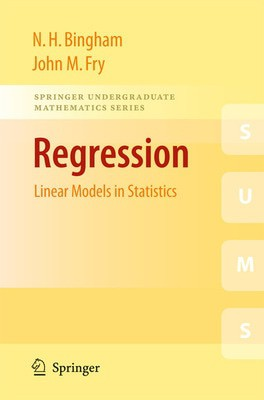Hurry, item low in stock!

# Regression - Nick BinghamHurry only 1 in stock!
FREE Delivery on ALL Orders!
Title
Regression - linear models in statistics
Author
Nick Bingham
format
Paperback / softback
Publisher
Springer
Language
English
UK Publication Date
20100929

Regression is the branch of Statistics in which a dependent variable of interest is modelled as a linear combination of one or more predictor variables, together with a random error.
The subject is inherently two- or higher- dimensional, thus an understanding of Statistics in one dimension is essential.

Regression: Linear Models in Statistics fills the gap between introductory statistical theory and more specialist sources of information. In doing so, it provides the reader with a number of worked examples, and exercises with full solutions.

The book begins with simple linear regression (one predictor variable), and analysis of variance (ANOVA), and then further explores the area through inclusion of topics such as multiple linear regression (several predictor variables) and analysis of covariance (ANCOVA). The book concludes with special topics such as non-parametric regression and mixed models, time series, spatial processes and design of experiments.

Aimed at 2nd and 3rd year undergraduates studying Statistics, Regression: Linear Models in Statistics requires a basic knowledge of (one-dimensional) Statistics, as well as Probability and standard Linear Algebra. Possible companions include John Haigh's Probability Models, and T. S. Blyth & E.F. Robertsons' Basic Linear Algebra and Further Linear Algebra.

##### We are Rated Excellent on TrustpilotHere's what you say about us...

From the reviews:

"The present book is intended for a second undergraduate or beginning graduate course in statistics providing further study of this single topic. … Complete, mathematically rigorous proofs are routinely provided for theorems. The fully-worked examples and solutions to the exercises are detailed. … Linear Models in Statistics is highly suitable for a theoretical statistics course for advanced undergraduate math majors, beginning math graduate students or others interested in using the book for independent study." (Susan D'Agostino, The Mathematical Association of America, December, 2010)

"Intended primarily for advanced undergraduate and beginning graduate students with knowledge of the basic concepts of statistics, probability, and linear algebra, this student-friendly book provides a lucid presentation of numerous regression analysis topics. … A salient feature is the numerous, carefully selected worked examples and complete solutions to all the problems in various chapters. Includes a useful index and bibliography. Summing Up: Recommended. Upper-division undergraduates, graduate students, and professionals." (D. V. Chopra, Choice, Vol. 48 (8), April, 2011)

"This book describes the linear regression statistical models as a core of statistics, from simple linear regression (with one predictor variable) and analysis of variance (ANOVA) to more extended topics as multiple linear regression (with two or more predictor variables) and analysis of covariance (ANCOVA). … The contents of the book are addressed in most part to the undergraduates students (but with some chapters appropriate for master level) having a basic knowledge of linear algebra, probability and statistics." (Nicoleta Breaz, Zentralblatt MATH, Vol. 1245, 2012)

Type
BOOK
Edition
2010
Keyword Index
Regression analysis.
Country of Publication
England
Number of Pages
284